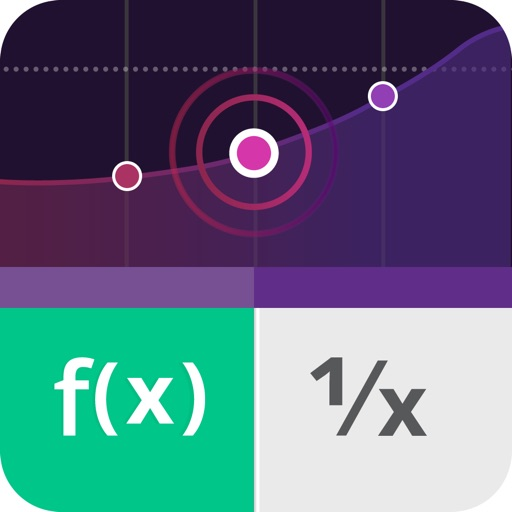Graphing Calculator50% OFF# Graphing Calculator

## Scientific Graphing Calculator

2.5.6 for iPhone, iPad
\$4.99 · \$9.99
9.6

12 Ratings

Size

53.0 MB

Graphing Calculator Description
Award winning Graphing Calculator app for iPhone and iPad. Designed with simplicity, usability and beauty in mind!

After installing Graphing Calculator+ you get access to 6 different calculators:

1- Graphing calculator
2- Handwriting calculator
3- Scientific calculator
4- Quadratic & Cubic equation solver
5- System of linear equations solver
6- Base Converter between HEX, OCT, BIN & DEC

FEATURES:
- Elegant & intuitive interface
- Supports handwriting
- Displays both the equation & the result at the same time
- Supports many scientific functions
- Solving system of linear equations
2x – y = 9
3x + 4y = –14
- Solving Quadratic equation
x² + 5x + 6 = 0
- Solving Cubic equation
2x³ - 4x² - 22x + 24 = 0
- Graph equations and find their local minimum, local maximum & intersection points.
Graphing Calculator 2.5.6 Update
2019-12-14 Version History
Bugs fixing and features enhancements
More